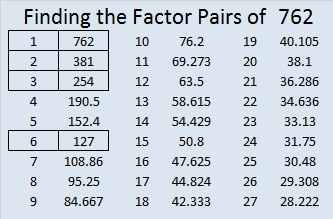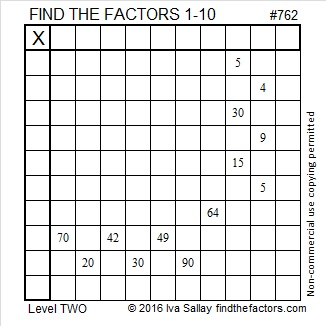# 762 and Level 2

• 762 is a composite number.
• Prime factorization: 762 = 2 x 3 x 127
• The exponents in the prime factorization are 1, 1, and 1. Adding one to each and multiplying we get (1 + 1)(1 + 1)(1 + 1) = 2 x 2 x 2 = 8. Therefore 762 has exactly 8 factors.
• Factors of 762: 1, 2, 3, 6, 127, 254, 381, 762
• Factor pairs: 762 = 1 x 762, 2 x 381, 3 x 254, or 6 x 127
• 762 has no square factors that allow its square root to be simplified. √762 ≈ 27.604347.This level 2 puzzle isn’t very difficult:Print the puzzles or type the solution on this excel file: 10 Factors 2016-02-04

—————————————

Here’s a little more about the number 762:

People who memorize the digits of pi have to stop someplace. Wikipedia informs us that one place to stop is known as the Feynman point which is 999999 beginning at pi’s 762nd decimal place. It is named after Richard Feynman who reportedly said in a lecture that he would like to recite from memory the digits of pi up to that point because he could then end the recitation by saying 999999 and so on. Perhaps he would even be able to make pi sound like a rational number? Be sure to check out the highlighted digits of both pi and tau that appear in a graphic in that article. There is also an explanation of how truly unusual a sequence of six repeating digits can be.

762 can be written as the sum of consecutive numbers three ways:

• 253 + 254 + 255 = 762; that’s 3 consecutive numbers. (254 × 3 = 762)
• 189 + 190 + 191 + 192 = 762; that’s 4 consecutive numbers.
• 58 + 59 + 60 + 61 + 62 + 63 + 64 + 65 + 66 + 67 + 68 + 69  = 762; that’s 12 consecutive numbers.

762 can also be written as the sum of two consecutive prime numbers: 379 + 383 = 762, and as the sum of four consecutive prime numbers: 181 + 191 + 193 + 197 = 762.

762 is the sum of three squares three different ways:

• 25² + 11² + 4² = 762
• 23² + 13² + 8² = 762
• 20² + 19² + 1² = 762

762 is palindrome and repdigit 222 in BASE 19 because 2(19²) + 2(19) + 2(1) = 762.

—————————————